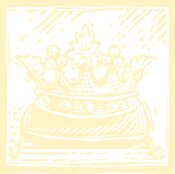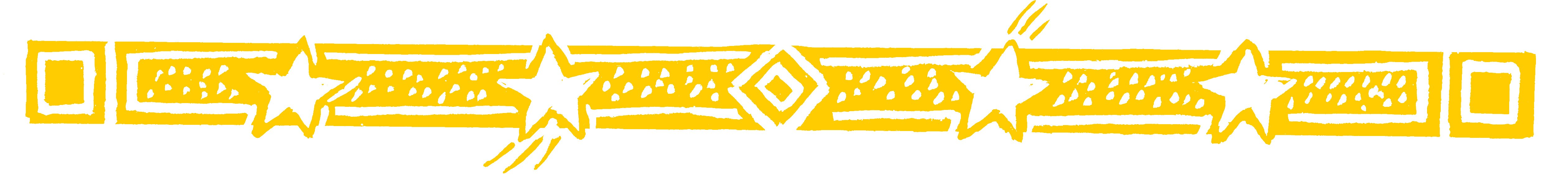IN THE DIY SHOPThis astonishing method for multiplying numbers is
so easy because it works from left to right

Suppose you want to know the cost of 6 hinges at 73 cents each.

• 73 × 6 = 438

We first multiply the 7 by 6 to get 42.

We then multiply the 3 by 6 to get 18.

4 2 18 = 4 3 8

The 1 in the 18 is mentally carried back to the 42
to make it 43.

So any double figure number after the first involves
carrying the first figure to the left and adding it on.

• Similarly 234 × 7 = 1638

We get 2×7=14 then 3×7=21
and 14 21 = 161

Next we have 4×7=28, so 161 28 = 1638

• 282 × 4 = 1128

As 8 32 = 112 and 112  8 = 1128

• And 43 × 7 = 2821 = 301 (28 becomes 30)

Question Comment !
 Tutorial Title Question Title No Questions 0 Questions Asked 0 Skipped Questions 0 Correctly Answered 0 Wrongly Answered 0 Total Question Attempts 0 Current Question Attempts 0

Skip Question

Reset Test

Halt Test

 No Questions 0 Questions Asked 0 Skipped Questions 0 Correctly Answered 0 Wrongly Answered 0 Total Question Attempts 0

Time Taken

CloseIn The DIY ShopMathematics is the
Queen of the Sciences
and Arithmetic the
Queen of Mathematics
GAUSS